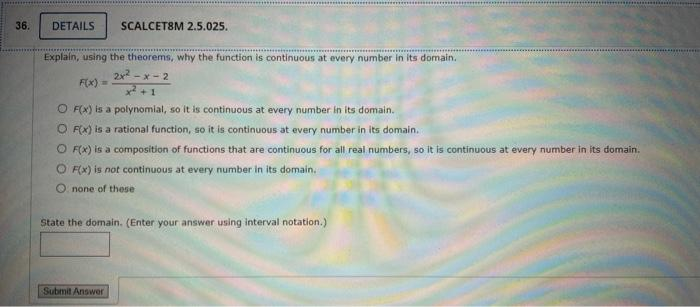### Create an Account

Home / Questions / DETAILS SCALCET8M 2.5.025. Explain, using the theorems, why the function is continuous at ...

# DETAILS SCALCET8M 2.5.025. Explain, using the theorems, why the function is continuous at every number in its domain. 2x²-x-2 F(x) = O F(X) is a polynomial, so it is continuous at every number in its

DETAILS SCALCET8M 2.5.025. Explain, using the theorems, why the function is continuous at every number in its domain. 2x²-x-2 F(x) = O F(X) is a polynomial, so it is continuous at every number in its domain. OFIX) is a rational function, so it is continuous at every number in its domain. O F(X) is a composition of functions that are continuous for all real numbers, so it is continuous at every number in its domain. O FX) is not continuous at every number in its domain. none of these state the domain. (Enter your answer using interval notation.) Submit AnswerJun 05 2021 View more View LessSubscribe To Get Solution